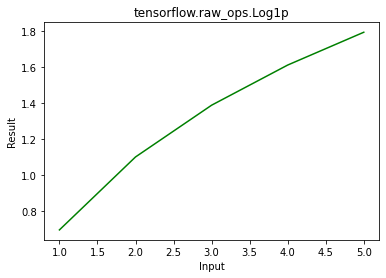Related Articles

# Python – tensorflow.raw_ops.Log1p()

• Last Updated : 05 Jun, 2020

TensorFlow is open-source python library designed by Google to develop Machine Learning models and deep learning  neural networks. TensorFlow raw_ops provides low level access to all TensorFlow operations. Log1p() is used to find element wise logarithm of (1+x) for input x.

Syntax: tf.raw_ops.Log1p(x, name)

Parameters:

• x: It’s the input tensor. Allowed dtype for this tensor are bfloat16, half, float32, float64, complex64, complex128.
• name(optional): It’s defines the name for the operation.

Returns:  It returns a tensor of same dtype as x.

Note: It only takes keyword arguments.

Example 1:

## Python3

 `# Importing the library``import` `tensorflow as tf`` ` `# Initializing the input tensor``a ``=` `tf.constant([``1``, ``2``, ``3``, ``4``, ``5``], dtype ``=` `tf.float64)`` ` `# Printing the input tensor``print``(``'Input: '``, a)`` ` `# Calculating logarithm(1 + x)``res ``=` `tf.raw_ops.Log1p(x ``=` `a)`` ` `# Printing the result``print``(``'Result: '``, res)`

Output:

```Input:  tf.Tensor([1. 2. 3. 4. 5.], shape=(5, ), dtype=float64)
Result:  tf.Tensor([0.69314718 1.09861229 1.38629436 1.60943791 1.79175947], shape=(5, ), dtype=float64)

```

Example 2: Visualization

## Python3

 `# importing the library``import` `tensorflow as tf``import` `matplotlib.pyplot as plt`` ` `# Initializing the input tensor``a ``=` `tf.constant([``1``, ``2``, ``3``, ``4``, ``5``], dtype ``=` `tf.float64)`` ` `# Calculating logarithm(1 + x)``res ``=` `tf.raw_ops.Log1p(x ``=` `a)`` ` `# Plotting the graph``plt.plot(a, res, color ``=``'green'``)``plt.title(``'tensorflow.raw_ops.Log1p'``)``plt.xlabel(``'Input'``)``plt.ylabel(``'Result'``)``plt.show()`

Output:Attention geek! Strengthen your foundations with the Python Programming Foundation Course and learn the basics.

To begin with, your interview preparations Enhance your Data Structures concepts with the Python DS Course. And to begin with your Machine Learning Journey, join the Machine Learning – Basic Level Course

My Personal Notes arrow_drop_up